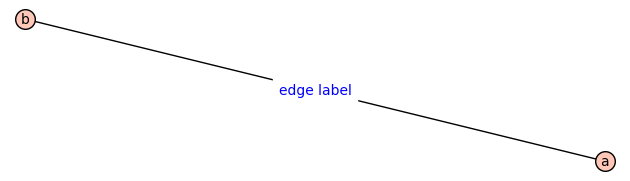# Changing display labels of nodes on a graphHi, I have created a graph which is in fact a tree. Each node has a label that I gave at creation time. Now, when I plot the graph for each node this "internal" label is displayed. Is it possible to have different display labels than this "internal" label?

Thanks

edit retag close merge delete

Can you provide a small example to illustrate what you have and what you want in a simple case?

The idea is the following. Let's imagine I have defined the graph : G2 = Graph([('a', 'b', 'edge label')]). Like that it is displayed with node labels 'a' et 'b'.

But I want to display G2 (as it is defined) but as G3 = Graph([('Good', 'Morning')]) will be displayed

The structure and meaning of your last sentence escape me.

Mmmh sorry for my bad english. I found finally another way to solve my problem, I think there was no solution to what I wanted to do. Thanks a lot for your time.

Sort by » oldest newest most voted

One possibility is that you are specifying the edge labels, not the vertex labels:

sage: G = Graph([('a', 'b', 'edge label')])
sage: G.plot(edge_labels=True)Since edge labels are not shown by default, what you see in G.plot() are the vertex labels only (that you call "internal").

more

Oh! yes good idea, if I remove the node labels and keep only edge labels can be a way to go. Thanks